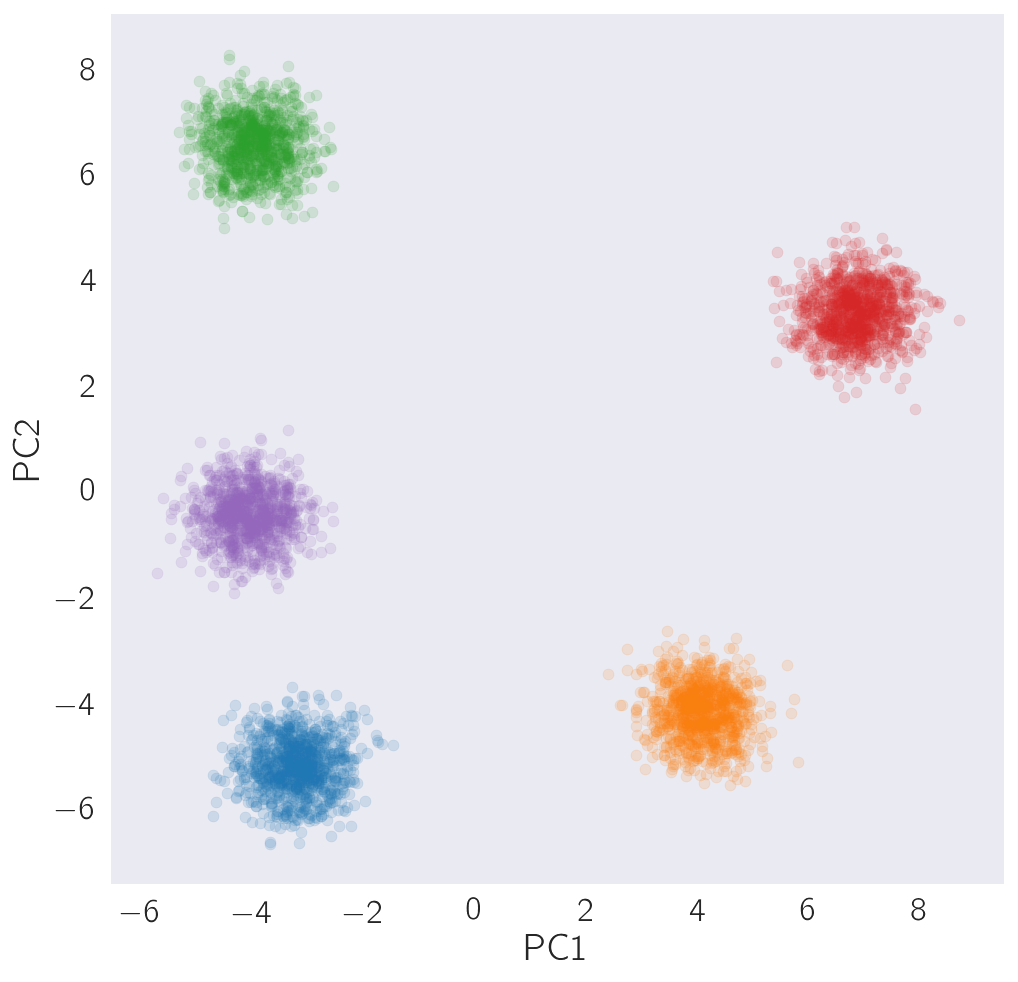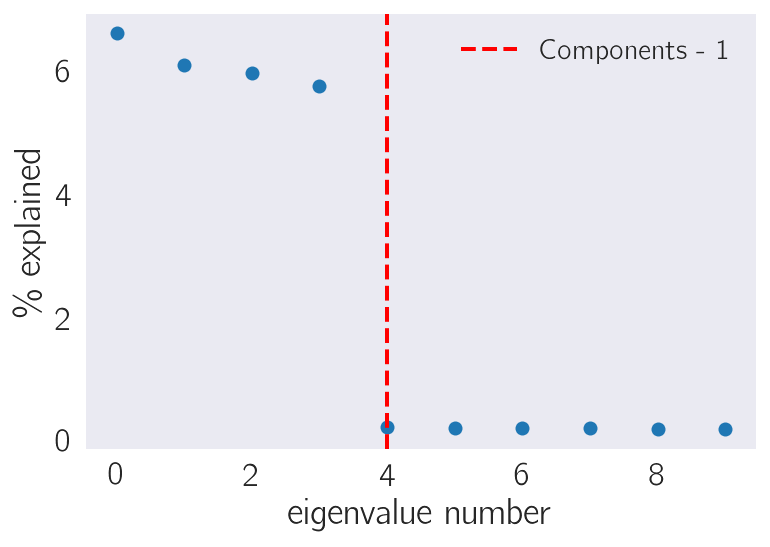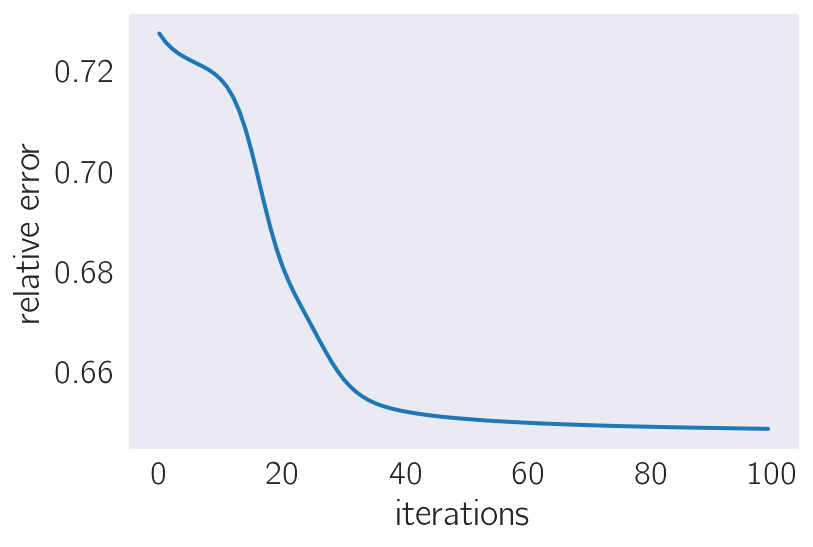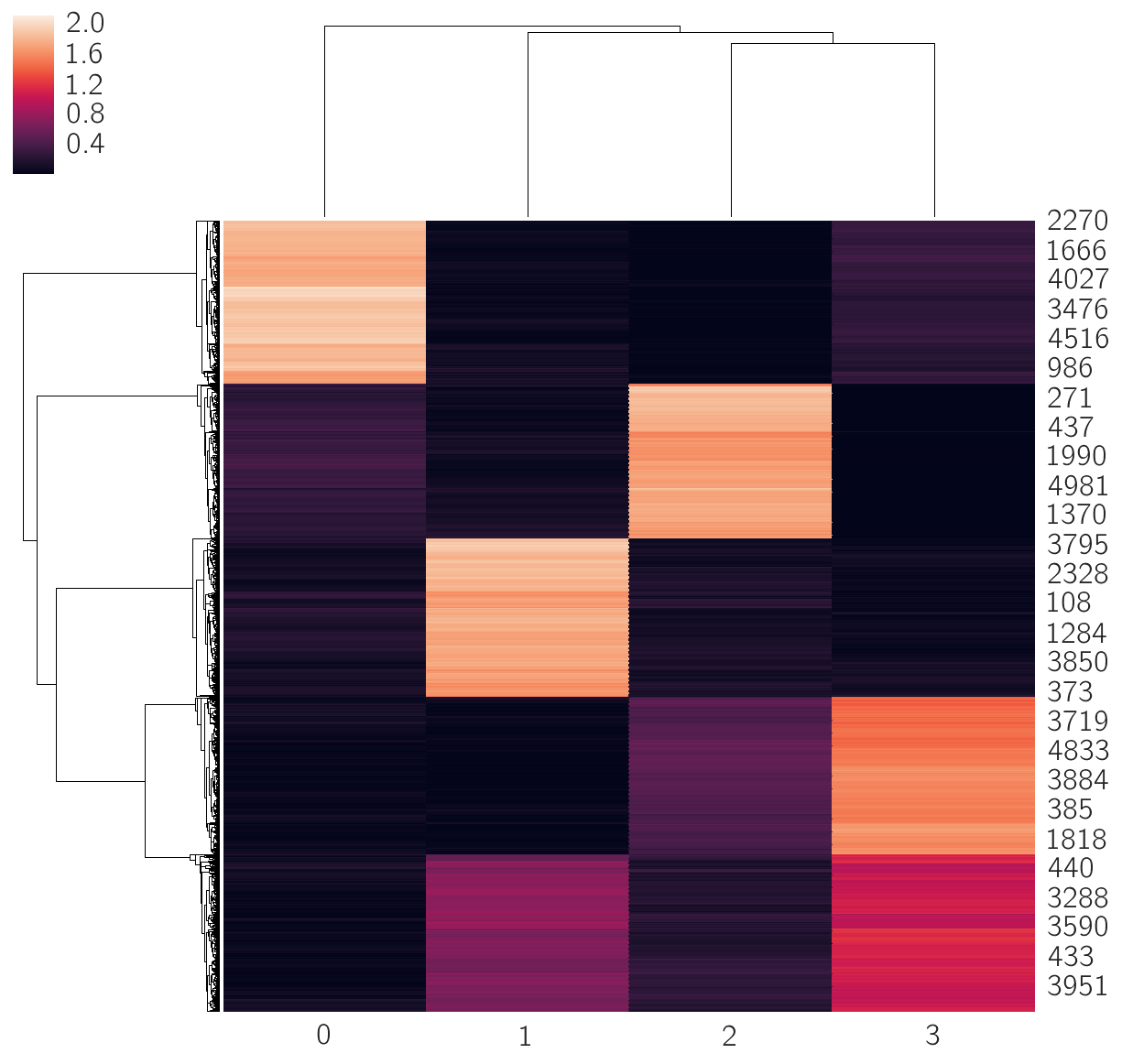# Non-negative Matrix Factorization¶

Non-negative Matrix Factorization is a dimensionality reduction approach that is growing in popularity in the bioinformatics community. Because it is a matrix factorization approach, it, like PCA, is a linear method and in this sense the results can be used to naturally cluster data.

The gist of NMF is as follows: given a (non-square) matrix $X$, find two matrices $W$ and $H$ such that their product minimizes the squared error, $||X - WH||_F^2$. If $X$ has dimensions $m \times n$, then it follows that $W$ must have dimensions $m \times k$ and $H$ must have dimensions $k \times m$. Thus, our algorithm must minimize the squared error for a given $k$ (which is to be specified by the user).

How do we perform such a factorization? One method is to initialize $W$ and $H$ randomly, then perform gradient descent on $W$ and on $H$. In other words, we would like to calculate: $$\nabla_W e = \nabla_W ||X - WH||_F^2 = \nabla_W Tr[(X^T - H^TW^T)\cdot(X - WH)].$$

At this point, I admit ignorance as to how the gradient operation is performed for a matrix. I understand gradients of a vector, but my matrix calculus is rusty, if ever I learned it. At any rate, the gradients can be written in a simple form: $$\nabla_W e = -XH^T + WHH^T,$$ and $$\nabla_H e = -W^TX + W^T WH.$$

We can update the matrix $H$ as follows: $$H_{ij} \leftarrow H_{ij} + \mu_{ij} \nabla_W e.$$

We can choose $\mu_{ij}$, the learning rate, in such a way that this updating requires multiplication of a single term, without addition,

$$\mu_{ij} = - H_{ij} \frac{(-W^T X)_{ij}}{(W^TWH)_{ij}}.$$

In this case, the updated $H_{ij}$ becomes $$H_{ij} \leftarrow H_{ij} \frac{(W^T X)_{ij}}{(W^TWH)},$$ and we can derive updates for $W_ij$ analogously: $$W_{ij} \leftarrow W_{ij} \frac{(XH^T)_{ij}}{(WHH^T)_{ij}}$$

## Multiplicative updating has a neat interpretation¶

The thing I find nice about this method is that the above equations are interpretable. For example, $W^TX$ can be viewed as a "reconstructed" $\hat{H}$. $W^TWH$ aims to represent the expected $W^T \widetilde{X}$, which is an "expected" $\widetilde{H}$. For $H_{ij}$ says that if "reconstructed" $\hat{H}_{ij}$ is smaller than the "expected" $\widetilde{H_{ij}}$, then the current entry $H_{ij}$ is currently too large and yields a bloated representation of $X$, so it should be shrunk.

The updating rule for $W_{ij}$ is analogous. The numerator calculates a reconstructed $W_{ij}$, whereas the denominator calculates a low-dimensional $\widetilde{X}$ and subsequently from this it calculates an "expected $\widetilde{W}_{ij}$. If the expected number is larger than the reconstructed number, then the current entry must be shrunk. Otherwise, it must be made larger.

# Implementation¶

In the code below, I implement a simple version of NMF, generate some fake data from five different clusters and use NMF to find $k$ clusters in the data, varying $k$.

In :
import pandas as pd
import numpy as np
import scipy as scipy
from sklearn.decomposition import PCA
import matplotlib as mpl
import matplotlib.pyplot as plt
import seaborn as sns
import altair as alt
from matplotlib import rc

# plotting settings
rc('text', usetex=True)
rc('text.latex', preamble=r'\usepackage{cmbright}')
rc('font', **{'family': 'sans-serif', 'sans-serif': ['Helvetica']})

%matplotlib inline

# This enables SVG graphics inline.
%config InlineBackend.figure_formats = {'png', 'retina'}

# JB's favorite Seaborn settings for notebooks
rc = {'lines.linewidth': 2,
'axes.labelsize': 18,
'axes.titlesize': 18,
'axes.facecolor': 'DFDFE5'}
sns.set_context('notebook', rc=rc)
sns.set_style("dark")

# more parameters
mpl.rcParams['xtick.labelsize'] = 16
mpl.rcParams['ytick.labelsize'] = 16
mpl.rcParams['legend.fontsize'] = 14


## Fake Data¶

Here, I will generate some fake data. To do this, I will, at random, generate $k$ vectors for which each coordinate can vary uniformly between 0 and 1. Then, I will at random generate $n$ samples. Each sample will choose a cluster to belong to at random. Next, I add gaussian noise to the samples, where the standard deviation of the noise at the $kth$ coordinate is equal to the cluster coordinate.

In :
dims = 1000  # dimensionality of the measurements
n = 5000  # number of points
components = 5  # number of components to generate data from
sigma = 3  # perplexity, lol

# generate component vectors to sample from
mu = np.random.uniform(0, 1, (dims, components))

# fake data
X = np.empty((n, dims))
clusters = np.empty(n)
for i in np.arange(n):
# pick a gaussian vector at random:
component = np.random.choice(components, 1)
mu_i = mu[:, component]
# generate noise in a poissonian fashion
noise = np.random.normal(0, mu_i)
obs = mu_i + noise
obs[obs < 0] = 0
# store
X[i, :] = obs[:,0]
clusters[i] = component


# Principal component analysis cleanly separates 5 clusters¶

In :
def plot_clusters(coords, n_clusters=4, cluster=np.array([]),
colorV=np.array([]), ax=None, **kwargs):
"""
Given a set of 2D coordinates, plot them in a single axes.
Params:
-------
coords - 2D coordinates
n_clusters -- number of clusters
colorV -- coloring for each cluster
ax -- provide an axis to plot into
**kwargs -- arguments for the ax.scatter function

Output:
------
ax - a matplotlib axis
"""
if not ax:
fig, ax = plt.subplots()

# go through each cluster
for i in np.arange(n_clusters):
# select the points in cluster i
x = coords[cluster == i, 0]
y = coords[cluster == i, 1]

# color the points according to the cluster color, or
# just go with the default seaborn color succession
if len(colorV):
color = colorV[cluster == i]
ax.scatter(x, y, label='Cluster {0}'.format(i), c=color, **kwargs)
else:
ax.scatter(x, y, label='Cluster {0}'.format(i), **kwargs)

# return
if not ax:
return fig, ax
else:
return None, ax


In the code below, I use the scikit.decomposition.PCA function on the data. The first two components separate the clusters quite nicely, even though they don't seem to explain very much of the variance.

In :
# normalize and pca
normX = (X - X.mean(axis=0))/X.std(axis=0)
pca = PCA(10)
coords = pca.fit_transform(X)

# plot
fig, ax = plt.subplots(figsize=(8, 8))
ax = plot_clusters(coords, n_clusters=components, cluster=clusters,
alpha=0.15, s=30, ax=ax)
_ = plt.xlabel('PC1')
_ = plt.ylabel('PC2')### The variance explained by the $n$th principal component drops off abruptly after $n$ exceeds the number of "effective" degrees of freedom in the dataset¶

In :
plt.plot(100*pca.explained_variance_ratio_, 'o')
plt.axvline(components-1, ls='--', color='red',
label='Components - 1')
_ = plt.legend()
_ = plt.xlabel('eigenvalue number')
_ = plt.ylabel(r'\% explained')# Implementation of the NMF algorithm.¶

The NMF algorithm occurs in two steps that resemble the Expectation-Maximization algorithm. Here, I code up those steps, and time them.

In :
def update_H(W, H, X):
"""
Update the H matrix based on the multiplicative method by Lee and Seung.

Params:
-------
W, H: NMF matrices
X: Data matrix

Output:
-------
newH: Updated H
"""
# initialize a new H
newH = np.empty(H.shape)
# calculate W.T * X (matrix multiply)
N = np.dot(W.transpose(), X)
# calculate W.T * W * H (matrix multiply)
D = np.dot(np.dot(W.transpose(), W), H)
# calculate H*N/D by element-wise multiplication
newH = np.multiply(H, np.multiply(N, 1/D))

return newH

%timeit update_H(W, H, X)

2.06 ms ± 367 µs per loop (mean ± std. dev. of 7 runs, 100 loops each)

In :
def update_W(W, H, X):
"""
Update the W matrix based on the multiplicative method by Lee and Seung.

Params:
-------
W, H: NMF matrices
X: Data matrix

Output:
-------
newH: Updated H
"""
# initialize a new W
newW = np.empty(W.shape)
N = X.dot(H.T)
D = W.dot(H).dot(H.T)
# element-wise multiply
newW = np.multiply(W, np.multiply(N, 1/D))
return newW

%timeit update_W(W, H, X)

16.8 ms ± 471 µs per loop (mean ± std. dev. of 7 runs, 100 loops each)


# Running NMF on the simulated dataset¶

Let's try running our NMF algorithm! It's not super-fast, but it's not bad for being implemented in about 10 lines of code. Let's guess there are $K=4$ clusters in the data and see what this looks like.

In :
def run_nmf(k, X, iters=100, verbose=True):
"""
A function to perform NMF using Lee and Seung's multiplicative method.

Params:
------
k: Number of clusters, integer
X: Data column, rows are observations, 2D numpy array or matrix
iters: Number of iterations to perform, integer
verbose: If true, prints the percent error rate every 10th iteration

Output:
W: The measurement by cluster matrix
H: The cluster by genes matrix
error: An array with the percent error at each iteration
"""
# normalize X
X = X/X.std(axis=0)

# if X has any negative entries, stop:
if (X < 0).any():
raise ValueError('X must be non-negative.')

n, dims = X.shape
# initialize W, the measurement by cluster matrix
W = np.random.uniform(0, 1, (n, k))
# initialize H, the cluster by genes matrix
H = np.random.uniform(0, 1, (k, dims))
# initialize a vector to store errors
error = np.empty(iters)

# iteratively update W and H
for i in np.arange(iters):
H = update_H(W, H, X)
W = update_W(W, H, X)

e = np.sqrt(np.sum((X - W.dot(H))**2))
e_rel = e/np.sqrt(np.sum(X**2))
error[i] = e_rel
if not verbose:
continue
if i % 10 == 0:
print('error is {0:.2f}'.format(e_rel))
return W, H, error

k = 4
W, H, error = run_nmf(k, X)

error is 0.73
error is 0.72
error is 0.68
error is 0.66
error is 0.65
error is 0.65
error is 0.65
error is 0.65
error is 0.65
error is 0.65


OK! We ran the algorithm for 100 iterations, and it seems to have converged fairly quickly. We can take a look at the percent error just to make sure:

In :
plt.plot(error)
plt.xlabel('iterations')
plt.ylabel('relative error')

Out:
Text(0,0.5,'relative error')Another thing we can do is take a look at the W matrix, which encodes the cluster-to-sample mapping.

In :
# plot what data points belong to what clusters
sns.clustermap(W)

Out:
<seaborn.matrix.ClusterGrid at 0x12bce2e80>Clearly, 4 clusters can separate the samples well. Curiously, there are some "cells" at the bottom right of the heatmap that look like they are expressing gene patterns that are intermediate levels of the gene regulatory networks that are expressed by the two parents. Note: Running the algorithm again results in slightly different answers, a sure indication that we are running into local, not absolute, minima, and that there are many of them. Fortunately, the clusters all look about the same size, and of similar intensity (save for a couple).

But wait. There were no parents. Those three clusters are not related, biologically or otherwise, in any way! What is going on? To figure it out, let's return to the PCA plot. This time, we will generate a PCA plot that is colored with a given column from the $W$ matrix. Let's see what we can find out.

In :
k=4

In :
def plot_pca_by_color(k, W):
# plot pca, coloring by the log of the weight values
fig, ax = plt.subplots(ncols=k, figsize=(5*k, 5))

for i, axis in enumerate(ax):
axis = plot_clusters(coords, n_clusters=components, cluster=clusters,
colorV=np.log(W[:, i]),
cmap='PiYG', alpha=0.15, s=30, ax=axis)
_ = ax[i].set_xlabel('PC1, $W_{0}$'.format(i))
_ = ax.set_ylabel('PC2')

return fig, ax

plot_pca_by_color(k, W)